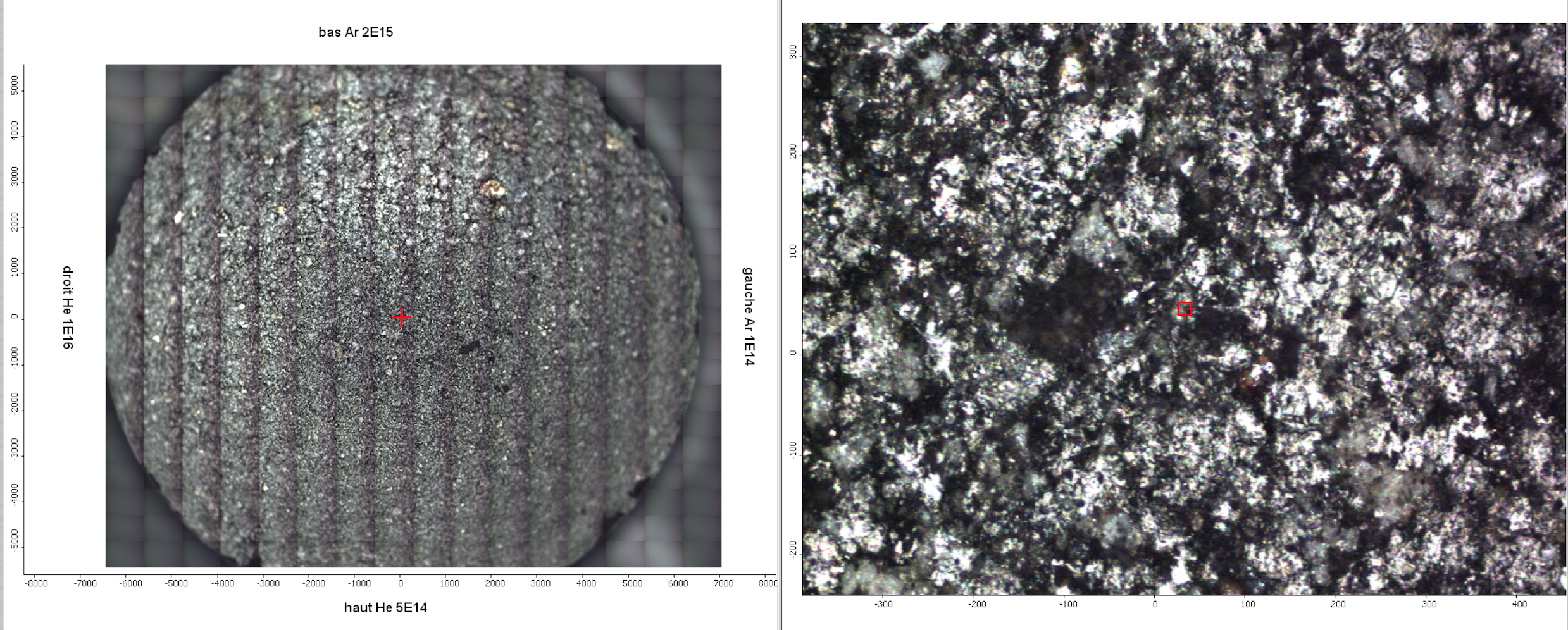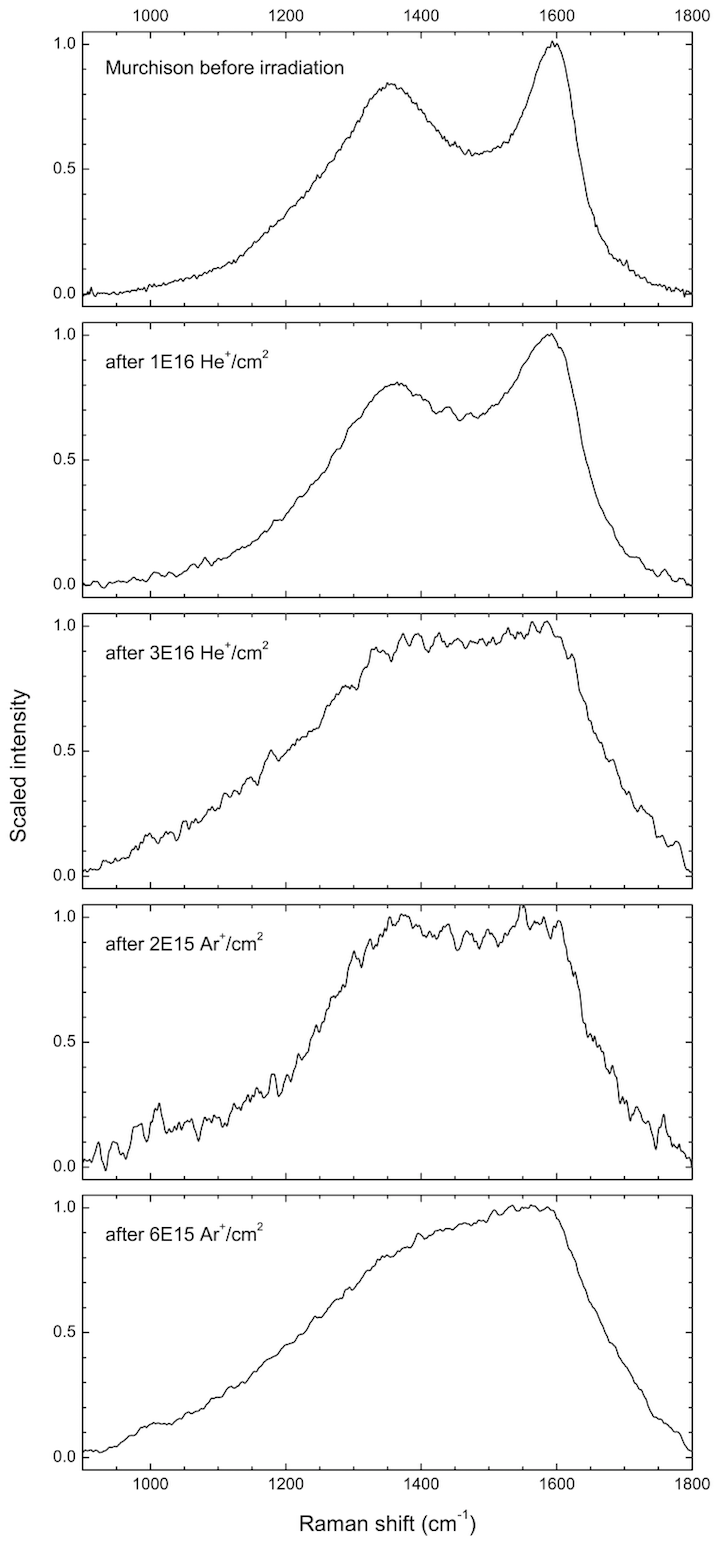Experiment
Title
Ion irradiation ($He^+$, $Ar^+$) of a Murchison meteorite pellets probed by Raman spectroscopy
Data reference
Brunetto, Rosario (2013): Ion irradiation ($He^+$, $Ar^+$) of a Murchison meteorite pellets probed by Raman spectroscopy. SSHADE/DAYSY (OSUG Data Center). Dataset/Spectral Data.
Publications
Database(s)
Experimentalists
Type(s)
laboratory measurement
Description
Raman spectra of pellets 1 and 2 of the Murchison meteorite, with different irradiation spots on the surface. Raman micro-spectroscopy was performed at the SMIS beamline at SOLEIL using a DXR Raman micro-spectrometer from Thermo Fisher with a 532 nm exciting laser radiation, and a power on the sample lower than 0.3 mW, producing power densities lower than 300 $W/mm^2$.
Number of spectra
6
Variable type(s)
We irradiated two pellets of Murchison using the SIDONIE isotope separator in CSNSM, Orsay, using $He^+$ and $Ar^+$ ions at 40 keV. Sample were mounted vertically and ion irradiation was performed at normal incidence. We produced four separate irradiation spots (4 mm in diameter each) on each pellet, using different fluences: $5~10^{14}, 2~10^{15}, 1~10^{16}, 3~10^{16}$ for $He^+$ ions.$cm^{-2}$, and $1~10^{14}, 5~10^{14}, 2~10^{15}, 6~10^{15}$ for $Ar^+$ ions.$cm^{-2}$. Earlier irradiated spots were masked to protect them from contamination of sputter deposits from the subsequent irradiation steps. A central area (diameter 2 mm) of each pellet remained non-irradiated.
Instrument
Thermo DXR - Raman
Sample holder
samples mounted horizontally on X-Y stage of microscope
Standard medium
vacuum
Observation mode
spectrum
Spectral range type(s)
MIR
Valid spectral range(s)
Min - Max ($cm^{-1}$) Sampling ($cm^{-1}$) Resolution ($cm^{-1}$) Position accuracy ($cm^{-1}$) Absorption edge
#1 902.0 - 1798.0 0.964 4.0 1.0
Raman shift with respect to laser at 532 nm
Confocal measurement with a x50 objective at long working distance
Observation mode
averaged
Spots number
50
Objective type
x50
Image size
1.0 x 1.0 ${\mu}m$